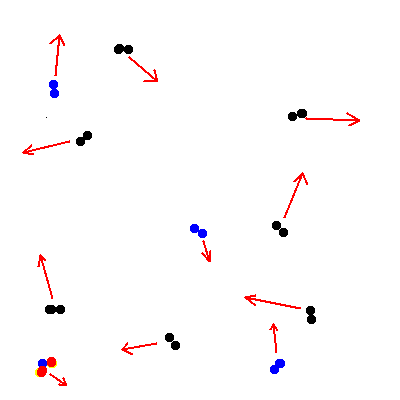# Escape velocity

## Whether a planet or moon can hold an atmosphere depends on how hot it is and how strong gravity is on the planet. Hot: If it's hot, the molecules of gas near the top of the atmosphere are bouncing around a lot. Sometimes they bounce right out into space, and if they are going fast enough they may not come back. So hotter means that the atmosphere may be lost -- especially the lighter elements.Gravity: The minimum velocity something has to have when leaving the surface of a planet in order to entirely escape from the planet is called the escape velocity. For example the escape velocity from Earth is about 11 km/sec. There is a simple relation: vesc2 = const. x M/R where M is the mass of the planet and R is its radius. (The constant is 2G where G is Newton's gravitational constant, but we won't use that.) We can conveniently use this by comparing to the earth. For example, if a planet has a mass equal to 2 times the mass of the earth and a radius equal to 3 times the radius of the earth, then (vesc)2 ~ 2/3 x (vesc,Earth)2 ~ 0.7 (vesc,Earth)2 An important example is the moon. The moon has a mass equal to (mass of Earth)/80 and a radius equal to (radius of Earth)/3 so (vesc)2 ~ 3/80 x (vesc,Earth)2 ~ 0.04 (vesc,Earth)2 The escape velocity from the moon is so much smaller than from the earth that it's no wonder that the moon can't keep an atmosphere! What if a planet had a mass equal to 10 times the mass of the earth and a radius of 3 times the radius of the earth. Would that planet do better or worse than the earth in holding an atmosphere?

Davison E. Soper, Institute of Theoretical Science, University of Oregon, Eugene OR 97403 USA soper@bovine.uoregon.edu